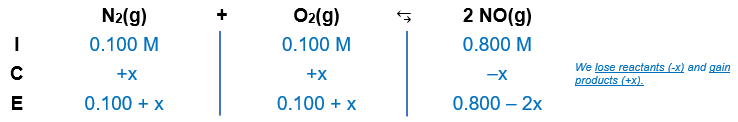# Problem: At equilibrium, the concentrations in this system were found to be [N2] = [O2] = 0.100 M and [NO] = 0.500 M. The balanced chemical equation is N2 + O2 ⇌ 2NO.If more NO is added, bringing its concentration to 0.800 M, what will the final concentration of NO be after equilibrium is re-established?

###### FREE Expert Solution

Given:

[N2] = [O2] = 0.100 M

[NO] = 0.500 M

Balanced chemical equation: N+ O⇌ 2NO

Step 1: Create an ICE chart.

• more NO added to a solution that was in equilibrium → system moves to the left side of the reaction to counteract the change

• the reverse reaction would be our basis for KcStep 2: Calculate K.

• the reverse reaction would be our basis for Kc

99% (213 ratings)###### Problem Details

At equilibrium, the concentrations in this system were found to be [N2] = [O2] = 0.100 M and [NO] = 0.500 M. The balanced chemical equation is N+ O⇌ 2NO.

If more NO is added, bringing its concentration to 0.800 M, what will the final concentration of NO be after equilibrium is re-established?